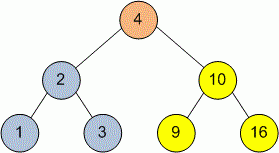Competitions

# Tree PostOrder Traversal

Implement a PostOrder traversal of a tree. In this traversal the left subtree is visited first, then the the right subtree and finally the root node.

Definition of a tree:

// Java
class TreeNode
{
public:
int val;
TreeNode left;
TreeNode right;
TreeNode(int x) {
val = x;
left = NULL;
right = NULL;
};

// C++
class TreeNode
{
public:
int val;
TreeNode *left;
TreeNode *right;
TreeNode(int x) : val(x), left(NULL), right(NULL) {}
};


Implement function PostOrder that prints in one line the vertices of the tree in order of PostOrder traversal. Separate the vertices with one space.

// Java
void PostOrder(TreeNode tree)

// C++
void Postrder(TreeNode *tree)


#### ExampleDuring the PostOrder traversal vertices will be printed in the next order: 1 3 2 9 16 10 4.

Time limit 1 second
Memory limit 128 MiB
Author Mykhailo Medvediev